# Rhodium oxide cluster

Structures and PBEPBE energies obtained so far. Energies marked with * have S2 more than 10 per cent out.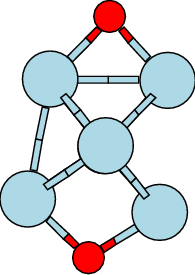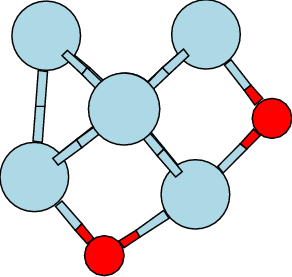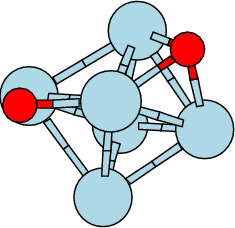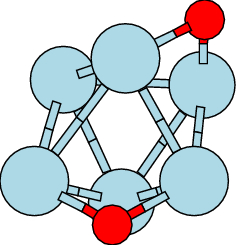8A E = -813.581592 Eh 8B E = -813.607070 Eh 8C E = -813.598333 Eh 8D E = -813.591212 Eh A ΔE = 0.69 eV B ΔE = 0.00 eV C ΔE = 0.24 eV D ΔE = 0.43 eV

Tri-oxides based on structure A

Structures and energies of different spin states of AA clusters. Little change in structure with spin state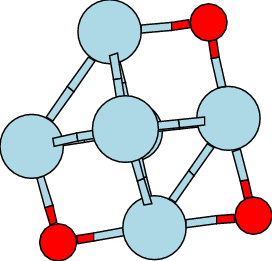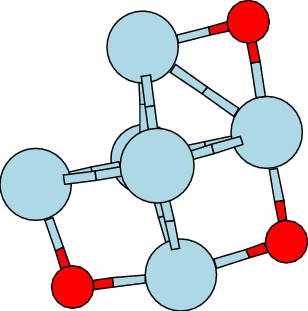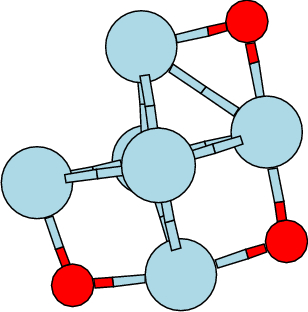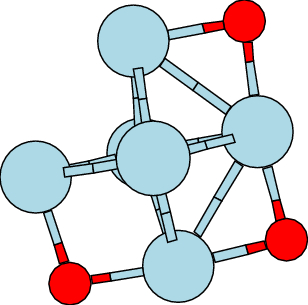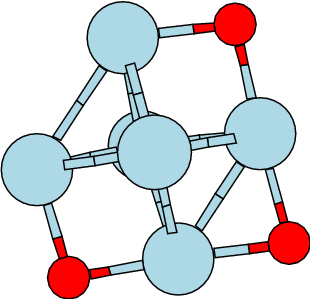2AA E = -888.775544 Eh 4AA E = -888.776034 Eh 6AA E = -888.780935 Eh 8AA E = -888.774400 Eh 10AA E = -888.763573 Eh

Structures and energeis of spin states of AB clusters. The distortion towards boat with higher spin cf the BB structures which go towards the boat at low spin.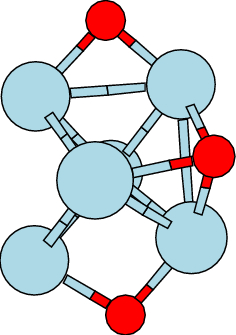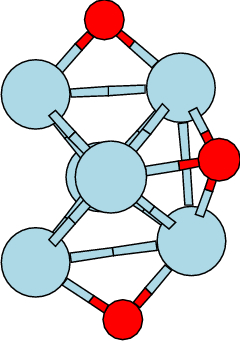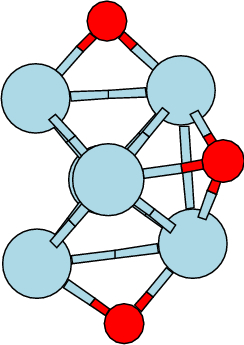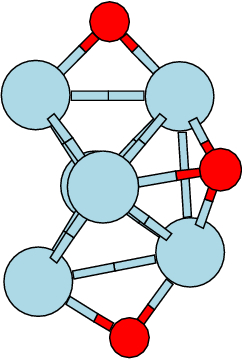2AB E = -888.762007 Eh 4AB E = -888.765134 Eh 6AB E = -888.767921 Eh 8AB E = -888.757827 Eh 10AB E = -888.745610 Eh

Tri-oxides based on structure B.

Energy and structure of different spin states of structure BB. Change from Oh- to boat-like structure of Rh6+.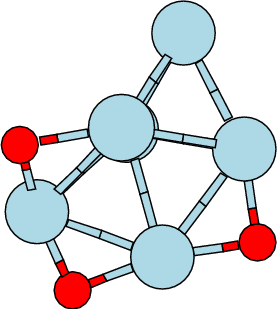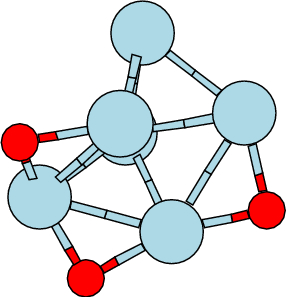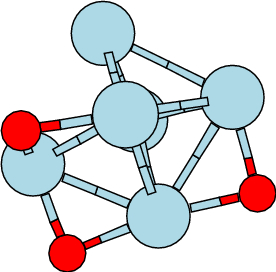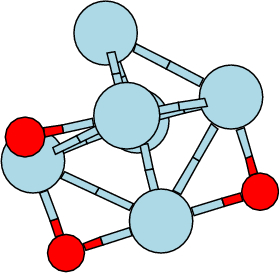4BB E = -888.782917 Eh 6BB E = -888.771490 Eh 8BB E = -888.761506 Eh 10BB E = -888.747109 Eh

Structure and energy of different spin states of structure BC. Change from distorted Oh- to CSP structure of Rh6+.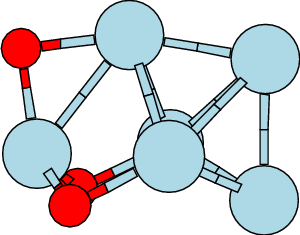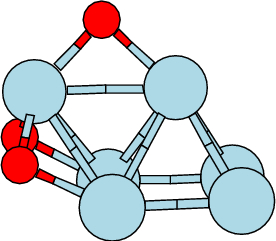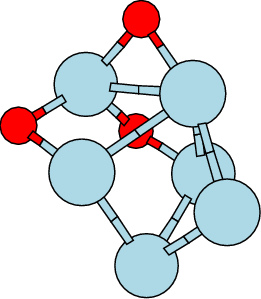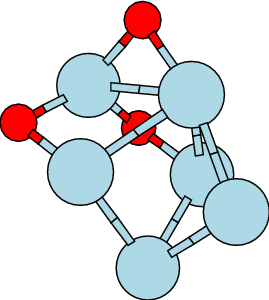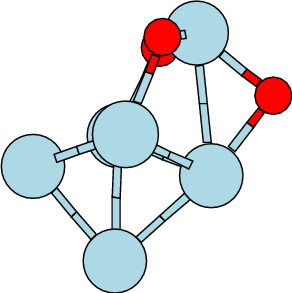2BC E = -888.759348 Eh 4BC E = -888.772168 Eh 6BC E = -888.756752 Eh 8BC E = -888.759647 Eh 10BC E = -888.744517 Eh S2 = 0.8 S2 = 3.77 S2 = 8.75 S2 = S2 =

Energy and structure of different spin states of structure BD.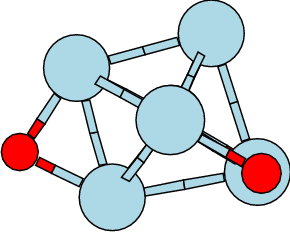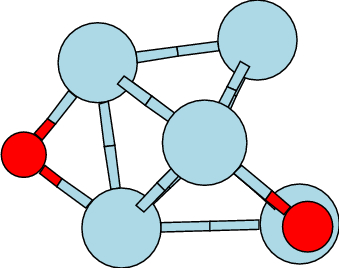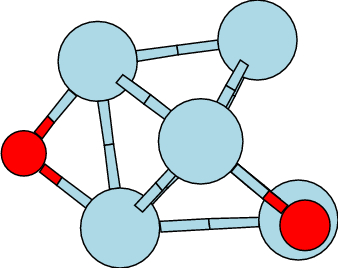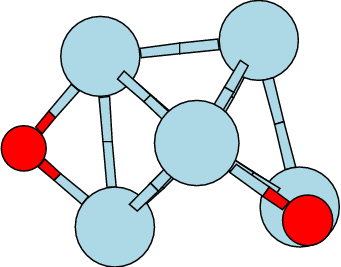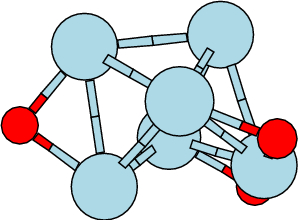2BD E = -888.766924 Eh 4BD E = -888.763595 Eh 6BD E = -888.765756 Eh 8BD E = -888.756697 Eh 10BD E = -888. Eh S2 = 1.0 S2 = 4.0 S2 = 8.75 S2 = S2 =

Tri-oxides based on structure C.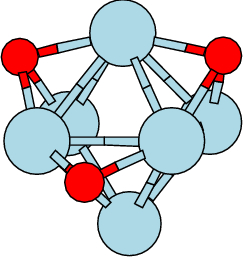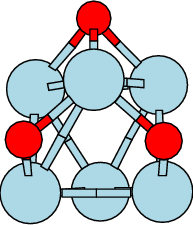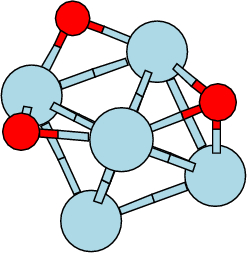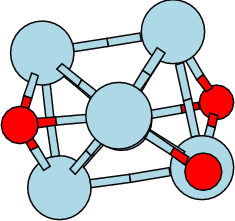2CA E = -888.776774* 2CB E = -888. Eh 2CC E = -888.776905* Eh 2CD E = -888.Eh 4CA E = -888.781785 4CB E = -888. Eh 4CC E = -888.780808 Eh 4CD E = -888.Eh 6CA E = -888.792832 Eh 6CB E = -888.745842 Eh 6CC E = -888.781158 Eh 6CD E = -888.766157 Eh 8CA E = -888.779846 Eh 8CB E = -888.742475 Eh 8CC E = -888.773538 Eh 8CD E = -888.763086 Eh 10CA E = -888.774175 Eh 10CB E = -888.741243 Eh 10CC E = -888.741243 Eh 10CD E = -888. Eh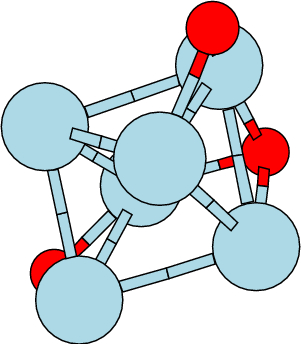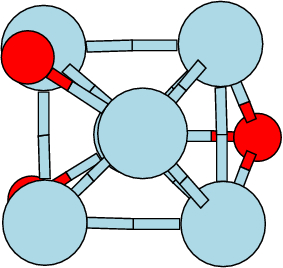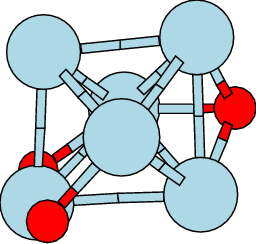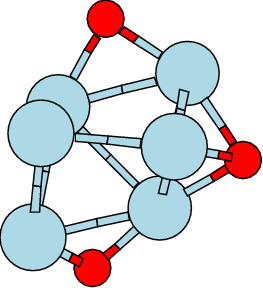2CE E = -888. 2CF E = -888. Eh 2CG E = -888. Eh 2CH E = -888.Eh 4CE E = -888. 4CF E = -888. Eh 4CG E = -888.7744825 Eh 4CH E = -888.Eh 6CE E = -888. 6CF E = -888. Eh 6CG E = -888.777487 Eh 6CH E = -888.Eh 8CE E = -888.757817 8CF E = -888.752343 Eh 8CG E = -888.771768 Eh 8CH E = -888.768957Eh 10CE E = -888. 10CF E = -888. Eh 10CG E = -888. Eh 10CH E = -888.Eh

Different spin states of structure CA. Changes in oxide binding site.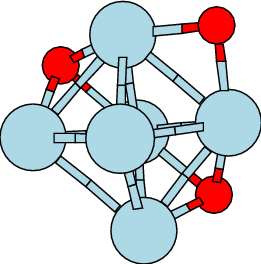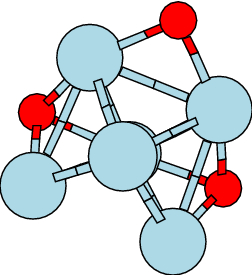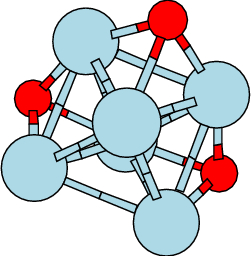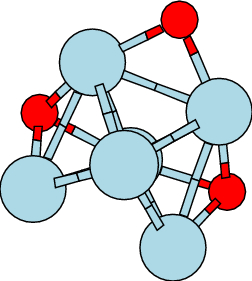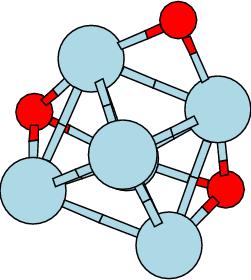2CA E = -888.776774 Eh 4CA E = -888.781785 Eh 6CA E = -888.792832 Eh 8CA E = -888.779846 Eh 10CA E = -888.774175 Eh S2 = 3.63 S2 = 3.8 S2 = S2 = S2 =

Structures based on di-oxide D.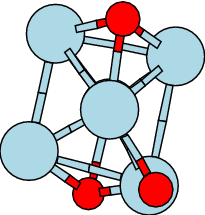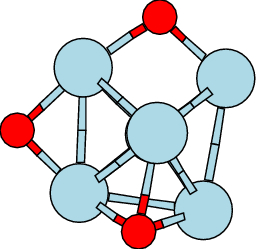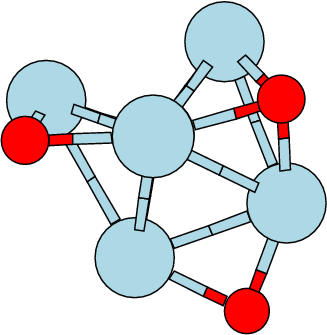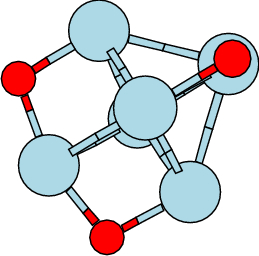8DA E = -888.762674 8DB E = -888.771981 Eh 8DC E = -888.760815 Eh 8DD E = -888.758658 Eh DA ΔE = eV DB ΔE = eV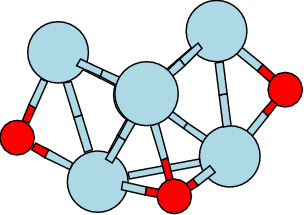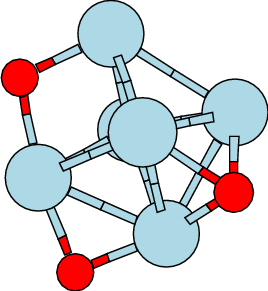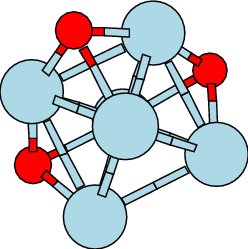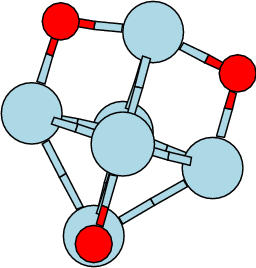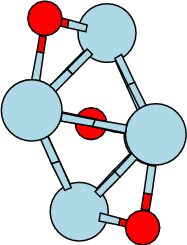2DE E = -888. Eh 2DF E = -888. Eh 2DG E = -888.780611* Eh 2DH E = -888. Eh 2DI E = -888. Eh 4DE E = -888.Eh 4DF E = -888. Eh 4DG E = -888.783724 Eh 4DH E = -888. Eh DI E = -888. Eh 6DE E = -888. Eh 6DF E = -888.777761 Eh 6DG E = -888.793033 Eh 6DH E = -888. Eh 6DI E = -888. Eh 8DE E = -888.757677 Eh 8DF E = -888.771829 Eh 8DG E = -888.783876 Eh 8DH E = -888.7758577 Eh 8DI E = -888.738596 Eh 10DE E = -888. Eh 10DF E = -888. Eh 10DG E = -888.778817 Eh 10DH E = -888. Eh 10DI E = -888. Eh